status
failed

# Learn to PID the Cart-Pole in the OpenAI Gym

This is a beginner’s introduction to PID controllers using the OpenAI gym. We’re going to build a PID controller and watch it work on the Cart-Pole system as simulated by the OpenAI gym project. For complete transparency, we’re only going to build a PD controller: we won’t use the integral term.

The Cart-Pole is a very simple robot. It’s a cart on a 1D track with a rod attached at the center which can swing around a pivot. The goal is to balance the pole upright. Here’s me trying to manually balance the pole by moving the cart left and right: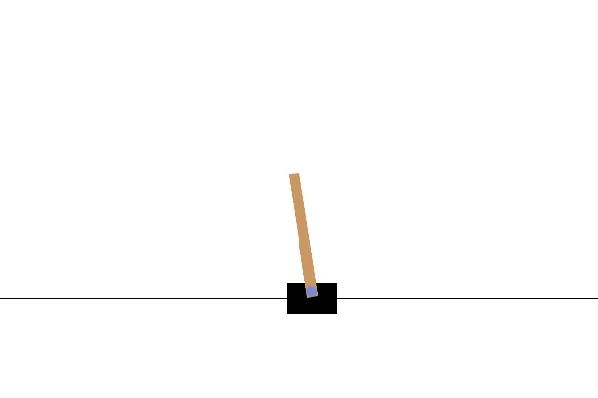As you can see, I’m pretty bad at it–notice that the “game” resets when the pole reaches a 90 degree angle. Here’s what the working controller will look like: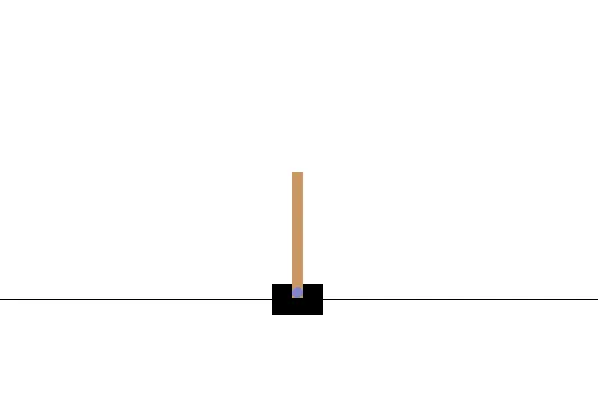Much better! Here’s the plan for how we’ll get there.

• Install OpenAI’s1 gym
• Play with the cart-pole system with your keyboard (as in the first picture)
• Implement a simple “intuitive” controller which doesn’t work very well
• Make PD controller using observations of the pole’s angle
• Combine two PID controllers based on the pole angle and cart position

The code for each step is also provided in this Git repository

If you don’t want to follow step by step, you can just skip the intro.

# Installing Gym and manually controlling the cart

To start, we’ll install gym and then play with the cart-pole system to get a feel for it. Create a virtualenv and install with pip:

python3 -m venv venv
source venv/bin/activate
pip install "gymnasium[classic_control]"

Now save the following code to a script, say play.py

#!/usr/bin/env python3
import gymnasium as gym
from gymnasium.utils.play import play
keys = { "a": 0, "d": 1 } # map keys 'a' and 'd' to actions left and right.
env = gym.make("CartPole-v1", render_mode="rgb_array")
play(env, keys_to_action=keys, noop=0) # noop=0 means default action is move left

and run it

> chmod +x play.py
> ./play.py

You should now be able to control the cart with your keyboard. Use a to move the cart left, and d to move right. You should see something like this: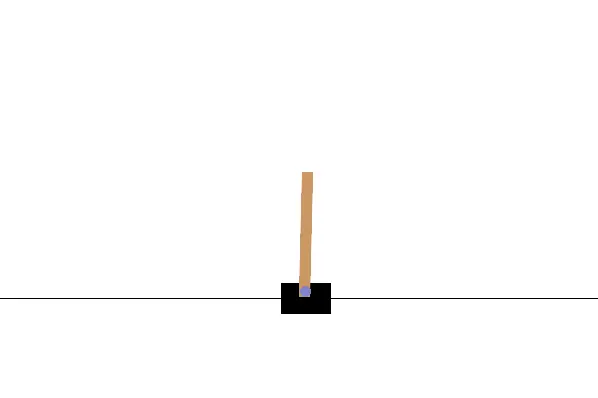If you’re on a tiling window manager and see a shrinking window, refer to this bug!

Notice that the default behaviour of Gymnasium is to reset the episode after the pole gets to only a 12 degree angle. This makes it a bit tough to play. If you want to make episodes last longer, you can just edit the environment installed in your virtualenv. Open the gymnasium cartpole file in your editor. For me, it’s here:

venv/lib/python3.10/site-packages/gymnasium/envs/classic_control/cartpole.py

… but this location will change based on your Python version. Now change this line:

self.theta_threshold_radians = 12 * 2 * math.pi / 360

to this:

self.theta_threshold_radians = 90 * 2 * math.pi / 360

which will reset the game only once the pole reaches a 90 degree angle.

# Building a simple controller

Before we build a PID controller, let’s just try something simple and see if it works. Now, the observations we get from the environment are 4-dimensional. We get the cart position, cart velocity, pole angle, and pole angular velocity. A simple thing to try is to keep the cart in the center by moving right when position is negative, and left when it’s positive. We can express this in code as follows:

#!/usr/bin/env python3
import gymnasium as gym

env = gym.make("CartPole-v1", render_mode="human")
observation, info = env.reset()
for _ in range(1000):
cart_position = observation
action = 1 if cart_position < 0 else 0
observation, reward, terminated, truncated, info = env.step(action)
if terminated or truncated:
observation, info = env.reset()
env.close()

This doesn’t really work, but it’s as good as I am: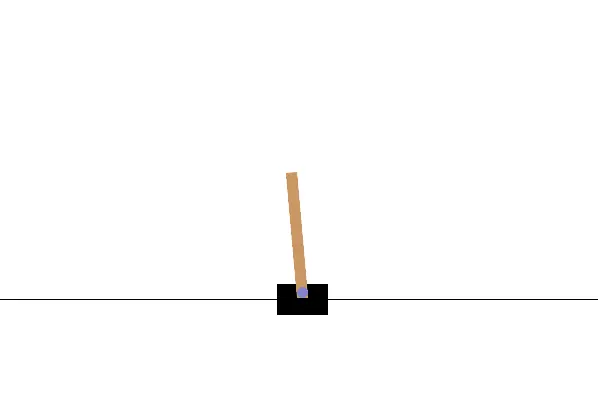You can find the code for this “controller” in simple_cart.py. What if instead we try keeping the pole upright instead of keeping the cart in the center? Change the action to reflect this:

pole_angle = observation
action = 1 if pole_angle > 0 else 0

and it behaves a little better…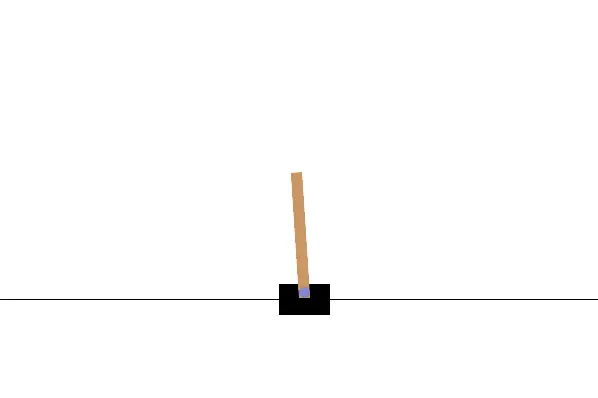… but still doesn’t quite solve the problem. The full code for this version is in in simple_pole.py.

# Proportional Derivative Control

What we made in the last section was actually a proportional controller, although it may not have looked like one. Let’s see what this means now.

Our “goal state” for the pole angle is 0, meaning the pole is balanced upright. A proportional controller applies a force to the cart which is proportional to the error: the difference between the goal state and the measured observation.

    error = goal - pole_angle

This error is multiplied by a constant $$k_p$$ to get the final control output.

    control_output = kp * error

However, since the action space of gymnasium’s cart-pole system is discrete, we can only choose to apply a fixed force either left or right. In the end, this amounts to simply taking the sign of control_output, which is exactly what we did in the previous section.

To make a better controller, we’re also going to have to add a derivative term to control_output.

Take another look at the behaviour of the simple_pole controller above.We can see that it starts off OK, but then starts oscillating out of control. We need something to “dampen” these oscillations, and this is exactly what the “derivative” term of a PD controller does. This is really beautifully explained here.

Our new control_output is going to be a weighted sum of error and the derivative of the error $$k_p \cdot e + k_d \cdot e'$$:

    control_output = kp * error + kd * d_error

Note that d_error can be computed completely numerically! I found this very confusing: there is no need to actually take a derivative here. You can just compute d_error using the previous value of the error:

    d_error = last_error - error

Here, last_error was the value of error at the previous timestep, so this approximates the rate of change of the error. To make this a bit clearer, let’s write the whole process down in code. You can find a complete script in pid_pole.py. Note also that I’ve given some values of $$k_p$$ and $$k_d$$ that work well enough, but actually finding these constants is out of scope for this blog post!

# The PD Controller

First, let’s create a class for the proportional-derivative controller described above.

class PD:
def __init__(self, kp, kd, goal):
self.kp = kp
self.kd = kd
self.goal = goal
self.last_error = 0

def observe(self, x):
error = self.goal - x
d_error = error - self.last_error
self.last_error = error
return self.kp * error + self.kd * d_error

We construct the class with constants constants $$k_p$$, $$k_d$$, and a desired goal state. Then in each time step of the simulation, we will call the observe function. This computes two things:

• error, the distance between the goal and our observation
• d_error, the difference between current and previous error

where the latter approximates the “rate of change” of the error. Finally, we save the error value, and return the control output corresponding to the expression $$k_p \cdot e + k_d \cdot e'$$.

We can use this simple controller in a script as follows:

controller = PD(kp=5, kd=100, goal=0)

env = gym.make("CartPole-v1", render_mode="human")
observation, info = env.reset()

for _ in range(1000):
pole_angle = observation
control_output = controller.observe(pole_angle)
action = 1 if control_output < 0 else 0

observation, reward, terminated, truncated, info = env.step(action)
if terminated or truncated:
observation, info = env.reset()

env.close()

Below is the controller in action. Find the complete script here.This is pretty good: the pole is balanced, and the oscillations are gone. However, we still have a problem: we aren’t trying to keep the cart in the center, and so it can start to drift away.

# Two PD Controllers

We’ll fix this drift issue by including a second PD controller for the cart’s position, and then just summing the control outputs. Let’s add a Controller class that combines two PD controllers.

class Controller:
def __init__(self):
self.cart = PD(kp=1, kd=100, goal=0)
self.pole = PD(kp=5, kd=100, goal=0)

def observe(self, cart_position, pole_angle):
u_cart = self.cart.observe(cart_position)
u_pole = self.pole.observe(pole_angle)
action = 1 if u_pole + u_cart < 0 else 0
return action

We can call this with a simple modification to our main script (find it in pid.py):

controller = Controller()

env = gym.make("CartPole-v1", render_mode="human")
observation, info = env.reset()

for _ in range(1000):
cart_position = observation
pole_angle = observation
action = controller.observe(cart_position, pole_angle)

observation, reward, terminated, truncated, info = env.step(action)
if terminated or truncated:
observation, info = env.reset()

env.close()

That gets us to our final controller, whose behaviour looks like this…… so we’re done! A working PID controller for the cart-pole system.

# Acknowledgements

I found a few resources very helpful in writing this blog post:

1. This project is now maintained by the Farama foundation↩︎